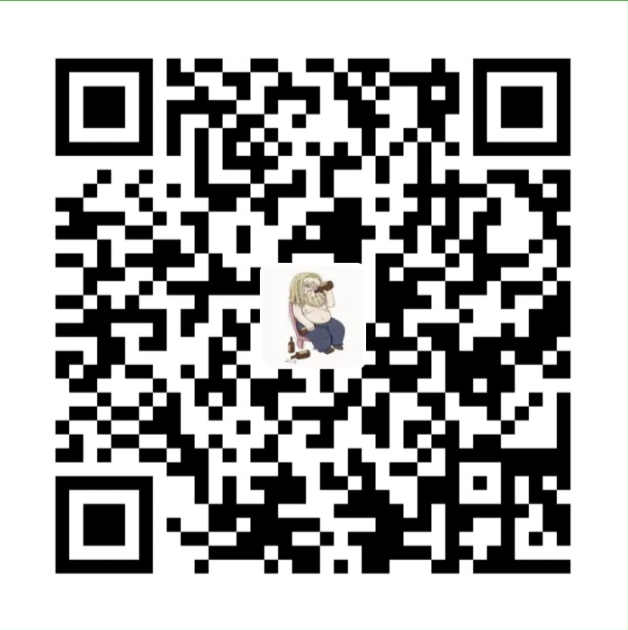• Rust中的每一个值都有一个对应的变量作为它的所有者。
• 在同一时间内，值有且只有一个所有者。
• 当所有者离开自己的作用域时，它持有的值就会被释放掉。

这里有两个重点：
• s 在进入作用域后才变得有效
• 它会保持自己的有效性直到自己离开作用域为止

``````let x = 5;
let y = x;``````

``````let s1 = String::from("hello");
let s2 = s1;

println!("{}, world!", s1);``````

``````let s1 = String::from("hello");
let s2 = s1.clone();

println!("s1 = {}, s2 = {}", s1, s2);``````微信支付宝